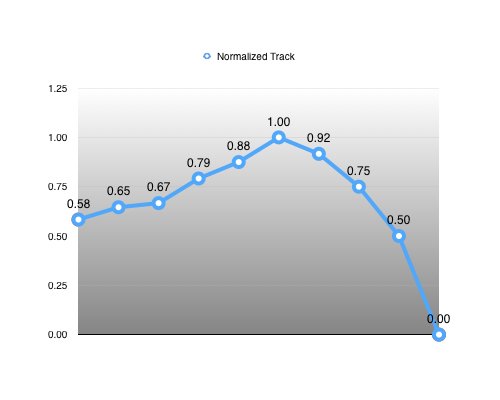# Animation Compression: Range Reduction

Range reduction is all about exploiting the fact that the values we compress typically have a much smaller range in practice than in theory.

For example, in theory our translation track range is infinite but in practice, for any given clip, the range is fixed and generally small. This generalizes to rotation and scale tracks as well.

# How It WorksUsing this track as an example, we first find the minimum and maximum value that will bound our range: `[16, 40]`

Using these, we can calculate the range extent: `40 - 16 = 24`

Now, we have everything we need to re-normalize our track. For every key: `normalized value = (input value - range minimum) / range extent`Reconstructing our input value becomes trivial: `input value = (normalized value * range extent) + range minimum`

We thus represent our range as a tuple: `(range minimum, range extent) = (16, 24)`

This representation has the advantage that reconstructing our input value is very efficient and can use a single fused multiply-add instruction when it is available (and it is on modern CPUs that support FMA as well as modern GPUs).

This increases the information we need to keep around by an extra tuple for every track. For example, a translation track would require a pair of Vector3 values (6 floats) to encode our 3D range.

This extra information allows us to increase our accuracy (sometimes dramatically). While both representations are 100% equivalent mathematically, this only holds true if we have infinite precision. In practice, single precision floating point values only have 6 to 9 significant decimal digits of precision. Typically, our original input range will be much larger than our normalized range and as such its precision will be lower.

This is most easily visible when our track needs to be quantized on a fixed number of bits. For example, our example translation track would typically be bounded by a large hardcoded number such as 100 centimetres. Our hardcoded theoretical track range thus becomes: `[-100, 100]`. Tracks with values outside of this range can’t be represented and might need a different base range or a raw un-quantized format. The above range is thus represented by the tuple: `(-100, 200)`. If we quantized this range on 16 bits, our input range of 200cm is evenly divided by 65536 which gives us a precision of: `200cm / 65536 = 0.003cm`. However, because we know the range is much smaller, using the same number of bits yields a precision of: `1.0 / 65536 = 0.000015 * 24cm = 0.00037cm`. Our accuracy has increased by 8x!

In practice, rotation tracks are generally bounded by `[-PI, PI]` but animated bones will only use a very small portion of that range on any given clip. Similarly, translation tracks are typically bounded by some hardcoded range but only a small portion is ever used by most bones. This translates naturally to scale tracks as well.

A side effect of range reduction is that all tracks end up being normalized. This is important for the simple quantization compression algorithms as well as when wavelets are used on an aggregate of tracks (as opposed to per track). Future posts will go further into detail.

# Conclusion

Range reduction allows us to focus our precision on the range of values that actually matters to us. This increased accuracy very often allows us to be more aggressive in our compression, reducing our overall size despite any extra overhead.

Do note that the extra range information does add up and for very short clips (e.g. 4 key frames) this extra overhead might yield a higher overall memory footprint. These are typically uncommon enough that it is simpler to accept that occasional loss in exchange for simpler decompression that can assume that all tracks use range reduction.

Up next: Uniform Segmenting